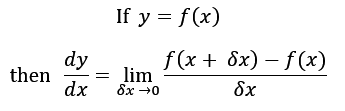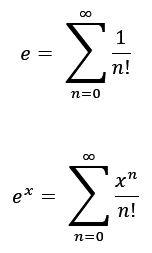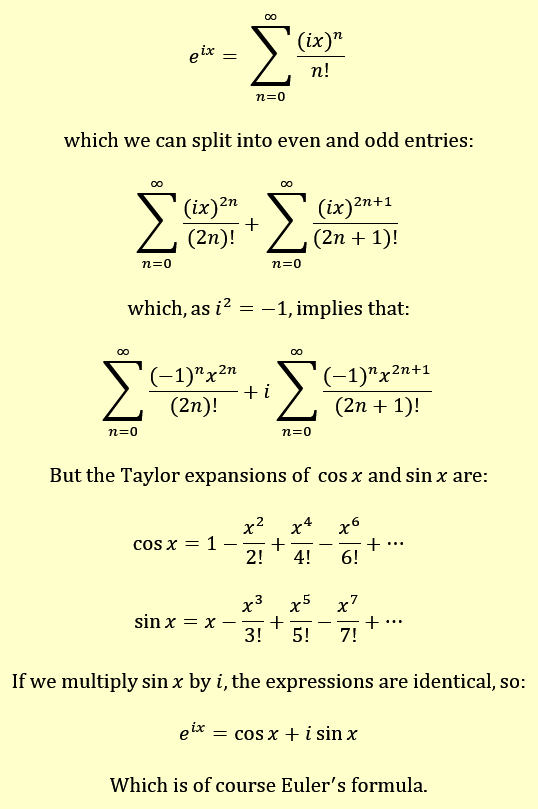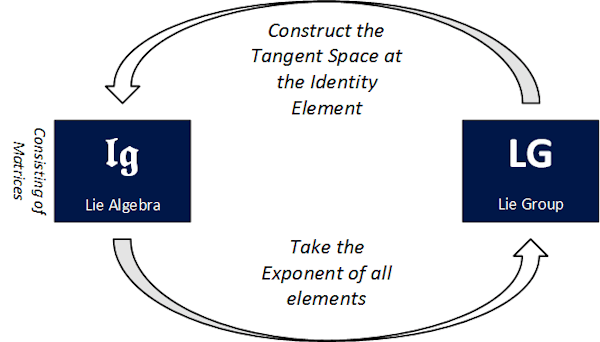# 20 – Power to Truth

 < ρℝεν | ℂσητεητs | ℕεχτ >“For since the fabric of the universe is most perfect and the work of a most wise Creator, nothing at all takes place in the universe in which some rule of maximum or minimum does not appear.” – Leonhard Euler

In Chapter 18, we introduced the concept of a Lie Algebra and, in Chapter 19, we defined a Lie Group and showed how the latter could be used to create the former via the procedure of constructing a Tangent Space. Later in this Chapter, we will look at the opposite side of this question, can we create a Lie Group from a Lie Algebra? However, having established at least a one-way connection already, we will begin by cataloguing some Lie Groups and their Lie Algebras.

The astute reader may have noticed that we have not really provided a mechanism for testing whether or not a specific Group is a Lie Group, i.e. whether it is also a differentiable manifold. This is intentional as a rigorous treatment would take some time. When considering matrix-based Groups, which is what we do a lot round here, it is helpful to note that most infinite matrix Groups will be Lie Groups; this includes all of the infinite matrix Groups we have met so far in this book. Below is a list of these types of Lie Groups (with a couple of gaps filled in, but still partial) together with their corresponding Lie Algebras :

 Lie Group Lie Algebra GLn(ℝ) The General Linear Group of degree n. The set of n × n Real invertible matrices. gln(ℝ) The Real Vector Space of n × n matrices with entries in ℝ and Lie Bracket being the commutator. This is equivalent to Mn(ℝ). SLn(ℝ) The Special Linear Group of degree n. The set of n × n Real invertible matrices with a determinant of 1. sln(ℝ) The Real Vector Space of n × n matrices with entries in ℝ, zero trace and Lie Bracket being the commutator. O(n) The Orthogonal Group of degree n . The set of n × n Real Orthogonal matrices, which describe both rotations and reflections of a body in n-dimensional Euclidean space. o(n) The Real Vector Space of n × n Skew-Symmetric matrices  with entries in ℝ and the Lie Bracket being the commutator SO(n) The Special Orthogonal Group of degree n. The set of n × n Real Orthogonal matrices with determinant equal to 1, which describe just the rotations of a body in n-dimensional Euclidean space. so(n) The Real Vector Space of n × n Skew-Symmetric matrices with entries in ℝ and the Lie Bracket being the commutator  U(n) The Unitary Group of degree n. The set of n × n Complex matrices for which their conjugate transpose is also their inverse. u(n) The Real Vector Space of n × n Skew-Hermitian matrices with entries in ℂ and the Lie Bracket being the commutator SU(n) The Special Unitary Group of degree n. The set of n × n Complex matrices for which their conjugate transpose is also their inverse and which have a determinant of 1. su(n) The Real Vector Space of n × n Skew-Hermitian matrices with entries in ℂ, zero trace and the Lie Bracket being the commutator Notes: The Lie Group of the first Lie Algebra we found, ℝ3 is ℝ3 itself, this is a general property of Euclidean space of any dimension. Obvious omissions from the above when considering Quantum Mechanics are the Spin Group and Symplectic Groups and their respective Lie Algebras. A more comprehensive list can be viewed on Wikipedia.

We have so far spoken about how a Lie Group can generate a Lie Algebra via constructing one of its Tangent Spaces. An obvious question is can we turn this round the other way? Can a Lie Algebra be used to generate a Lie Group? As it turns out this is a non-trivial question. The answer is easiest to describe for those Lie Algebras that are based on matrices; fortunately these are just the type of Lie Algebras that we need for our purposes. For matrix-based Lie Algebras we can give a concise answer and also demonstrate a mapping that generates a Lie Group from the set of matrices. However the actual process is not that simple to comprehend and requires us to spend some time considering something that was briefly referenced in both Chapter 11 and Chapter 13, the exponential function.

There and Back AgainHere I will return to our example of U(1), the Circle Group, and its Lie Algebra, u(1), the set of purely imaginary numbers. If we were to start with the Lie Algebra, how would we go about reconstructing the Lie Group? So we are looking for a mapping, φ:

φ: u(1) ↦ U(1)

or equivalently:

φ: {ai, a ∈ ℝ} ↦ {x + yi, x, y ∈ ℝ and x2 + y2 = 1}

When we last looked at the Circle Group, we noted that we could also express its members as eiθ and this gives us a clue as to how to proceed, let’s try the following definition of φ:

φ: u(1) ↦ U(1), ai ↦ eai

That is we map members of u(1) to their exponents.

Based on our work back in Chapter 13, it is pretty evident that this function will generate all elements of U(1) from members of u(1). Surely the above result is something of a one-off, depending on properties of unit circles and so on? If we take exponents of members of a general Lie Algebra, this won’t in general generate the related Lie Group, will it? Also what on Earth is meant by raising something to the power of a matrix anyway?

The answers to these questions, at least for Lie Algebras that consist of matrices, are “No, remarkably it’s not a fluke”, “Yes, exponentiating will generate the required Lie Group ” and “Please read on to find out”. In order to proceed, we first need to cover some more ground relating to the exponential function, including presenting a rigorous definition, one which we can repurpose to meet our needs.

What difference does it make?

When we referenced the exponential function back in Chapter 13 we made a distinction between an exponential function, y = ax, and the exponential function, y = ex. Here a is any fixed number, whereas e is a very special number, which is approximately equal to 2.71828 . The way that e is often introduced to schoolchildren is via the Calculus and specifically differentiation . Here we will do the same, with the motivation that the path we adopt is somewhat analogous to the one that Sophus Lie took in developing his Groups and Algebras; more on this later.

In the last Chapter, we mentioned that differentiation is effectively working out the slope of something, here we will focus on 1D curves for simplicity’s sake. If a curve is defined by some function, f(x), then rather than simply working out the slope at a point, differentiation will look to establish a second function, let’s call it f′(x), which captures the slope of f(x) everywhere. So f′(1) will be the slope of the function f at the point x = 1, f′(89.34) is the slope of f at the point x = 89.34 and so on.

The notation f′(x) itself is often used for the differential, but more commonly we make explicit what is being differentiated using one or other of the following notations:We read the first formulation as the differential of f(x) with respect to x and the second as the differential of y with respect to x; the latter recalls our convention of a functions input values being plotted on the x-axis and its output values, f(x) on the y-axis.

To give some examples:

 f(x) f′(x) or df(x)/dx ax + b a i.e. the slope of a line is a constant ax2 + bx + c 2ax + b i.e. the slope of a quadratic equation is a linear equation xn nxn-1 i.e. the slope of a polynomial of degree n is a polynomial of degree (n – 1) sin x cos x cos x – sin x

A linear equation represents a straight line  and a straight line has a constant slope. So the differential of a linear equation emerges naturally. With non-linear curves, i.e. ones which will have slopes that vary, it helps to define what we mean by the slope at a point.In the previous Chapter, we said that a plane could be a good estimate for a small segment of a sphere. In much the same way, in the above diagram, the slope of the red triangle is a good estimate for the slope at point x. What is more, as we make the value δx smaller, the estimate improves. It is this process, in concert with the concept of a limit, which both Newton and Leibniz used to define the differential of a function, f(x). A modern way of writing their definition is:The slope of our red triangle is, by definition, its height divided by its width, so:

Height of red triangle = f(x + δx) – f(x)

Width of red triangle = (x + δx) – x = δx

So slope = ( f(x + δx) – f(x) ) / δx

The idea of a limit is to take the statement “as we make the value δx smaller, the estimate improves” and push it to its logical conclusion when δx is is infinitely small, or infinitesimal . When this happens, rather than having a good estimate for the slope, the answer is the actual slope at x itself. This is what is meant by the “lim δx → 0” part of the definition above . It is by using the above definition that the values for f′(x) can be calculated.

So enough of our differentiation primer. What does this mean for our number e? Well the way that e emerges from this picture is that:

If f(x) = ex

then

f′(x) = ex

i.e. the function that captures the slope of ex at any point is ex itself.

e is unique in this respect, no other number has this property. In general:Where (using the convention employed in Pure Mathematics ) log(x) implies the natural logarithm of x. Natural logarithms are logarithms base e, so we have:

If a = eb

then

log a = b

Of course this means that when a = e, log a = log e = 1 and we get the differential that we started with.

 Note: It may occur to the reader that the process of differentiation can be extended. For a function f(x) with derivative f′(x), f′(x) is itself a function and may be capable of being differentiated to give us f′′(x). This is called a second order differential and is written d2f(x)/dx2. The classic (no pun intended) example here is that if f(t) is the displacement of a particle moving in space (where t stands for time), then f′(t) is its velocity (how fast the displacement is changing) and f′′(t) is its acceleration (how fast its velocity is changing). Depending on the properties of the function being considered, we can go on to form third, fourth and nth order differentials. Here we can offer a more rigorous definition of smoothness – a smooth function (which may of course delineate a smooth surface, or analogues thereof in higher dimensions) is one for which all orders of differentials exist. That is we can form dnf(x)/dxn for any n ∈ ℕ.

In SummarySo that was no doubt either illuminating, or at least a reminder of a few basic Mathematical facts. What does it have to do with our work creating Lie Groups from Lie Algebras. Well we have seen that the mapping:

φ: u(1) ↦ U(1), ai ↦ eai

generates the Lie Group U(1) from the Lie Algebra u(1). We have also said that using the exponential function will generate the Lie Group associated with a matrix-based Lie Algebra in general. One question that arose before is captured in the following exhibit:The above formulation may seem counterfactual, but equally we have been happily using things like eai to date and in many ways these are equally odd. For integers the meaning of ex is clear enough, it means multiply e by itself x times, so:

e2 = e × e

e5 = e × e × e × e × e

and so on.

We may even be happy to use results like:

xaxb = xa + b

which implies that:

x1/2x1/2 = x1/2 + 1/2 = x1 = x

which in turn means that:

√x = x1/2

Results like this point the way to other fractional powers of e and other numbers. But how do we rationalise something like e raised to the power of the square root of minus 1, let alone e to the power of some matrix?

The answer is to take a different and in many ways more robust definition of e, one that employs another kind of limit, this time the sum of an infinite series. An infinite series is something like:

a1 + a2 + a3 + …

Where the terms go on for ever.

Of course, depending on what values the ai take, we may often get a total figure of infinity. However, in some cases, the sum converges to a definite limit, the same way that ( f(x + δx) – f(x) ) / δx converges to the slope at x as δx tends to zero.

For example, one of the best known results is that:Where … implies that the sum goes on for ever.

People sometimes get confused by the above. If we pick the first n terms of the sum, we can get as close an approximation to 1 as we like. Pick a small number, say 0.0000000000001, and if I chose a big enough n, the difference between the first n terms of the sum and 1 will be less than the number you have picked. If you instead pick 0.000000000000000000000001, then a bigger value of n will suffice to get closer than this to 1. However, the whole infinite sum is not just close to 1, it is precisely 1, they are the same thing expressed in two different ways; the same way that 1, 1.0, 23/23, i4 and 0.999… are the same thing expressed five different ways.

In the last Chapter we made use of the summation symbol, a large sigma, which helps us to write infinite series more economically, using this notation our sum above can be written as:We can use the same approach to develop a better definition of e itself and also the function y = ex. First we will have to recall some notation from Chapter 3. This was the factorial of a number, n, written n!, where:

n! = 1 × 2 × 3 × … × (n – 1) × n

So

8! = 1 × 2 × 3 × 4 × 5 × 6 × 7 × 8 = 40,320

Using our sigma notation and factorial symbol (and also noting that 0! is defined as 1) we can make two definitions:The latter clearly reduces to the former where x = 1.

We are quite happy multiplying i by itself as many times as we like and also adding numbers including i, indeed we have been adding a lot of Complex Numbers recently, so the above definition allows us to define ei and other esoteric numbers. The following box uses this approach to establish the verity of Euler’s formula, as used extensively in Chapter 11.

 Euling the WheelsWhat is of more import to us here is that the series formulation of the exponential function, ex allows us to define what it means to raise e to the power of a matrix. Let’s pick a random matrix M, then we define the exponent of M as follows:As the above involves nothing more than multiplying matrices together, dividing them by integers and adding up the result (albeit doing all of these an infinite number of times), it can be seen that the definition works to extend ex to the realm of matrices. We should of course note that for terms like M2 to work, M must be a square matrix, but so are all the matrices in the Lie Algebras we have been dealing with. Also, in the same way that x0 = 1 for numbers, for matrices, M0 is the identity matrix of whatever size is relevant (shown as I above).

This means that we can finally state the following:

Consider a generic Lie Algebra lg , a generic element of which is a square matrix, M. The Lie Group, LG, generated by lg is the set:i.e. each matrix, M, in lg gets mapped to eM.

So, with a bit less hand waving than accompanied the journey from Lie Group to Lie Algebra, we have made the return trip back to a Lie Group, demonstrating the deep linkages between these constructs. The relationships that we have established are as summarised in the following diagram :An obvious question will be, what is the benefit of linking a Lie Group to a Lie Algebra? One answer is the type of phenomenon behind many such changes of perspective in Mathematics, Lie Algebras may allow easier calculation than Lie Groups and some results may be easily shown for a Lie Algebra when they are harder, or even out of reach for a Lie Group. We will discuss the motivations behind both Lie Groups and their related Lie Algebras further in the next Chapter. However Chapter 21 will commence by leveraging the work we have in recent Chapters to offer a different perspective on a Lie Group we have met before. This is our missing element from the formula SU(3) × SU(2) × U1(1), namely SU(3).

 Concepts Introduced in this Chapter Differentiation as a Limit The process of determining the slope at a point of a curve y = f(x) can be carried out as follows:This formula also provides a robust definition of a differential for curved surfaces and more complex objects. Generalised Exponential Function Both Euler’s number and the generalised exponential function are is defined via the sum of an infinite series as follows:Lie Algebra to Lie Group correspondence If a Lie Algebra, lg, consists of matrices, then the corresponding a Lie Group, LG, is (at least partially) reconstructed from lg by applying the exponential function to all elements of the Lie Algebra:< ρℝεν | ℂσητεητs | ℕεχτ >

Chapter 20 – Notes

  I should probably approach my naming more rigorously here, so SO(n) should probably be SOn(ℝ) or SO(n, ℝ) making clear both the degree of the Group and what Field is used for matrix entries. However I hope that the nomenclature is clear enough for our purposes.  While we have looked at both Orthogonal and Special Orthogonal Groups in Chapter 6, we have not derscribed their Lie Algebras before now.  Skew-symmetric matrices are ones whose transpose is its negative (AT = – A), so like Skew-Hermitian, but without the complex conjugate element.  No that is not a typo, o(n) and so(n) are the same thing. Put another way, the Tangent Spaces of O(n) and SO(n) are the same.  Or at least something ranging between the whole Lie Group and a significant sub-group, see Note 14.  Like π, e can be represented by a non-terminating, non-repeating decimal expansion, which starts 2.718 281 828 459 045 235 360 287 471 352 662 49… but never finishes.  The other point of entry is compound interest. If interest on an account is a rather generous 100% per annum and this is compounded annually, then the sum at the end of the year will be (1 + 1) of the principal, or two times. If it is compounded semi-annually, then it will be (1 + 1/2)2 (approximately 2.04414 times), quarterly and (1 + 1/4)4 (approximately 2.56578 times). If this halving of the period over which interest is compounded is continued, then after the (n-1)th halving of the compounding period the generic principal plus interest will be (1 + 1/2n)2n. If I state that if interest is compounded 220 times annually (i.e. just over a million times), then the principal plus interest after 12 months is about 2.71828. The limit of this sequence generates e as well.  The redundancy in this statement is appreciated.  We shall meet the word “infinitesimal” again before long.  I’m being rather non-rigorous here again, but to do otherwise would require a lot of other definitional work upfront. This is a book on Group Theory and neither Calculus nor Analysis, so I’ll stick to my non-rigorous statement, which does at least have the benefit of being valid.  Elsewhere ln is used to denote natural logarithms.  The type of rearrangement of terms in an infinite series that I am doing here is dependent on the series being absolutely convergent. You can prove almost anything by playing around with the terms of series that are not absolutely convergent.  Which we may note is normally named after its Lie Group, rather than the other way round.  The eagle-eyed among you at this point may raise an objection. As o(n) and so(n), the Lie Algebras of O(n) and SO(n) respectively have been stated to be the same thing, let’s call it so(n), then how can so(n) exponentiate to form both SO(n) and O(n), the former of which is an absolute sub-group of the latter? Well it can’t of course. For some Lie Groups exponentiating their Lie Algebra reconstructs all of the Lie Group, for others it reconstructs just part of it. In general the term is that “the local structure is recovered”. In the case of O(n) the matrices with a negative determinant are missing from the exponentiated Lie Algebra. The reasons for this are beyond the scope of this book.
 Text: © Peter James Thomas 2016-17. Images: © Peter James Thomas 2016-17, unless stated otherwise. Published under a Creative Commons Attribution 4.0 International License.